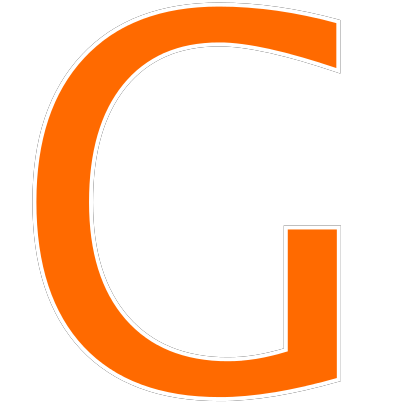# ATM Pin Puzzle Answer (SOLVED) | A House Wife Forgot Her Bank ATM Pin

ATM Pin Puzzle Answer, ATM Pin Code Puzzle is the latest puzzle that is making rounds on Facebook, Twitter, Whatsapp and more. The puzzle says that A housewife forgot her bank ATM pin, which is a four-digit number. But Luckily she remembered some hints on how to recall the pin. In this puzzle, you have to find the four-digit ATM pin code number.

So here we are with the ATM Pin Puzzle Answer. So now let us get started.

## ATM Pin Puzzle

ATM Pin Code Puzzle:

A housewife forgot her bank ATM pin, which is a four-digit number. Luckily she remembered some hints on how to recall the pin. The clues were that the first digit is half of the 2nd. The sum of 2nd and 3rd is 10. The 4th is equal to the 2nd plus 1. The sum of all the digit is 23. What is the ATM pin?

Related Puzzles:

iii i Over Two Circles Puzzle

Shoes Man Sunglasses Puzzle

Muscle Freak Maths Puzzle

A Pregnant Woman Goes To The Fridge Riddle

Here we are going to solve the ATM pin code puzzle and find the answer from the given clues.

Clues:

1. The clues were that the first digit is half of the 2nd.
2. The sum of 2nd and 3rd is 10.
3. The 4th is equal to the 2nd plus 1.
4. The sum of all the digit is 23

Solution:

As per the clue 1, the first digit is half of the 2nd. This also means that the 2nd digit is double of the 1st digit.

So let’s assume that the first digit of the atm pin is x.

Therefore the second digit is 2*x = 2x

The 2nd clue says the sum of 2nd and 3rd is 10.

So now let’s do the math:

2x + 3rd digit = 10

Therefore 3rd digit  = 10 – 2x

Now the 3rd clue says 4th is equal to the 2nd plus 10.

So the 4th digit = 2nd digit + 1 = 2x + 1

Now we have all the 4 digits of the atm pin. 1st is x, 2nd is 2x, 3rd is 10-2x & 4th is 2x+1.

As per 4th clue the sum of all the 4 digits are 23.

So,

• x + 2x + (10-2x) + (2x+1) = 23
• 3x + 11 = 23
• 3x = 23 – 11 = 12
• x = 12/3 = 4

Therefore,

1st digit = x = 4

2nd digit = 2x = 8

3rd digit 10 – 2x = 10 – 8 = 2

4th digit 2x + 1 = 8 + 1 = 9

Hence the ATM pin puzzle answer is 4829